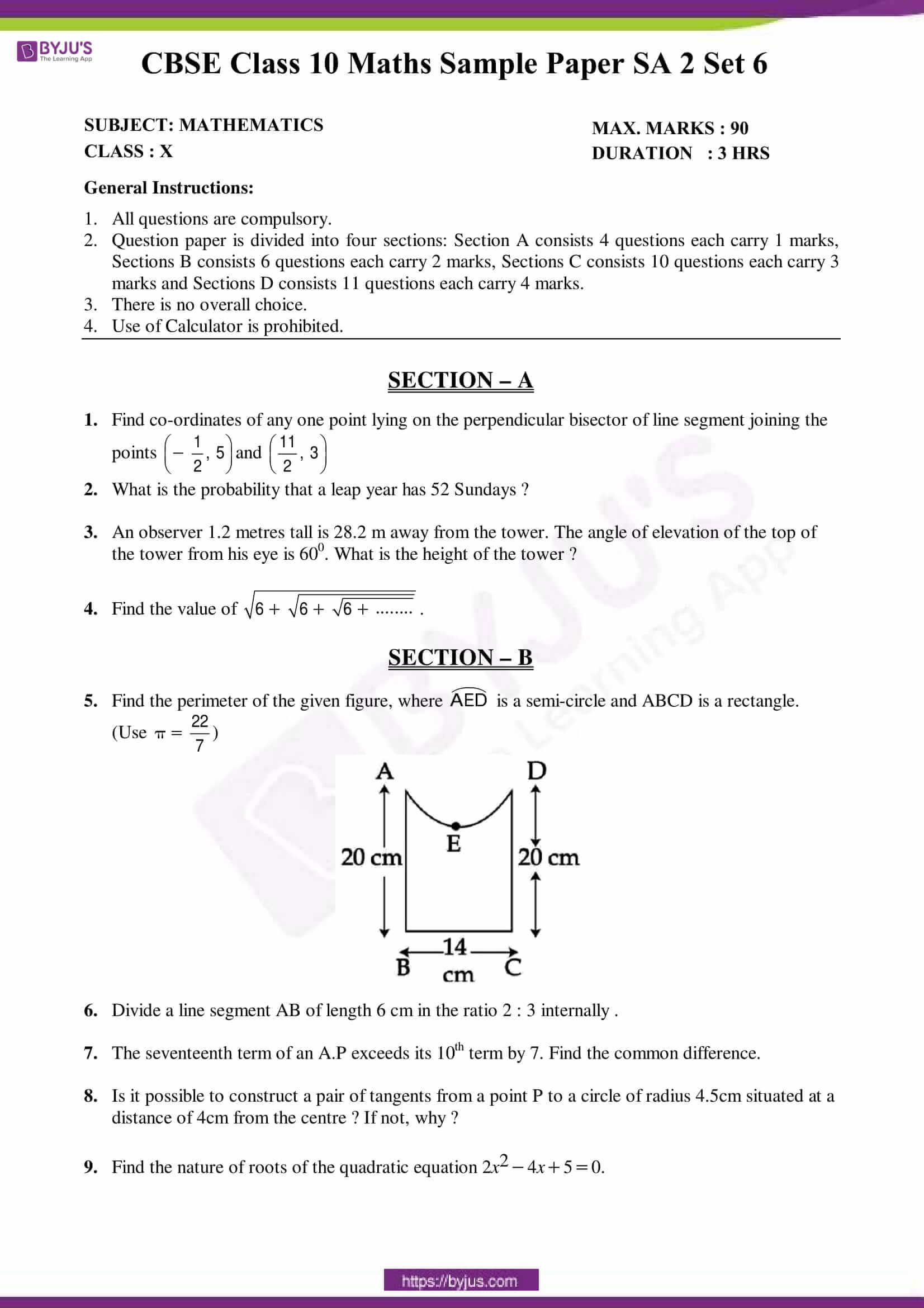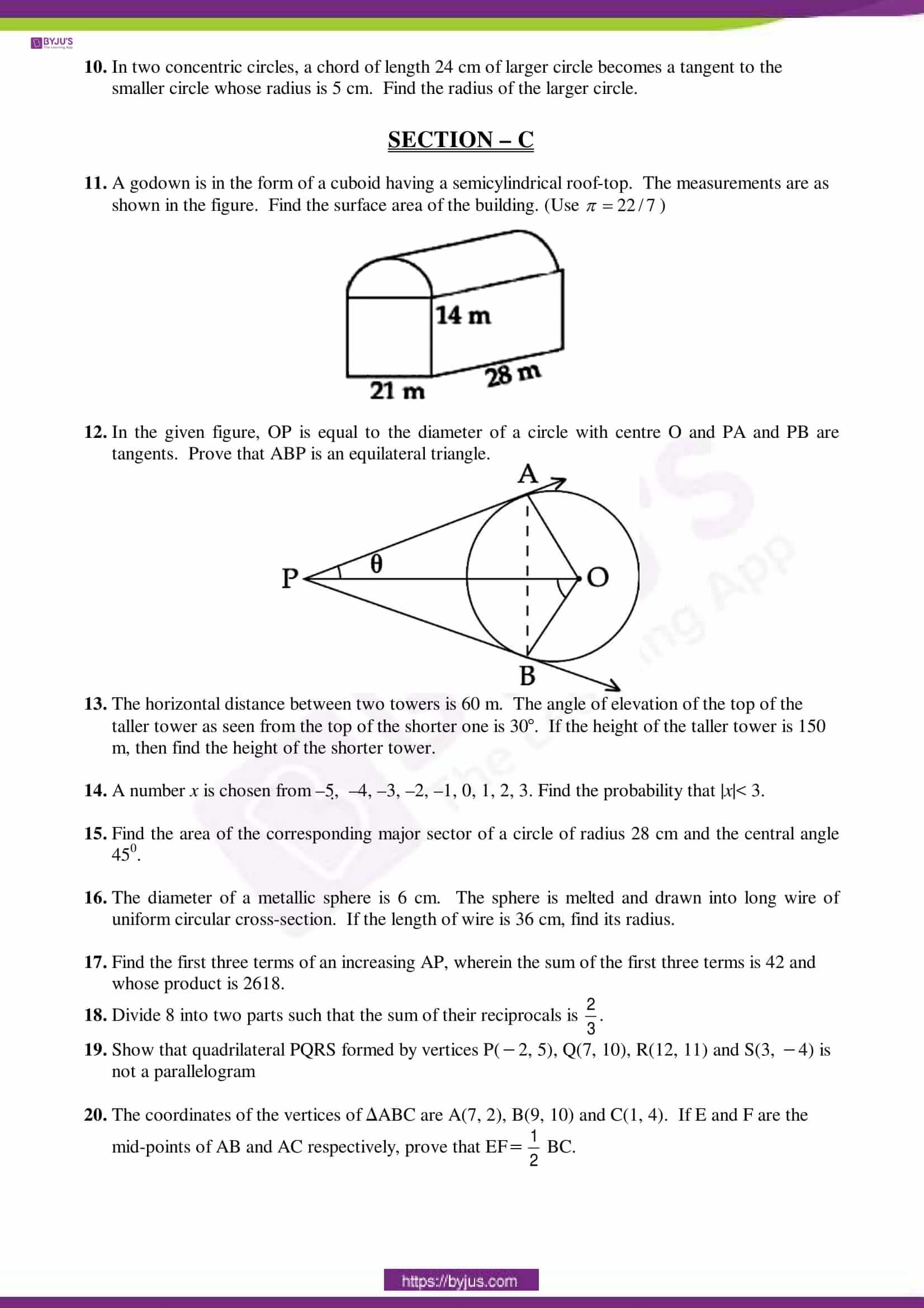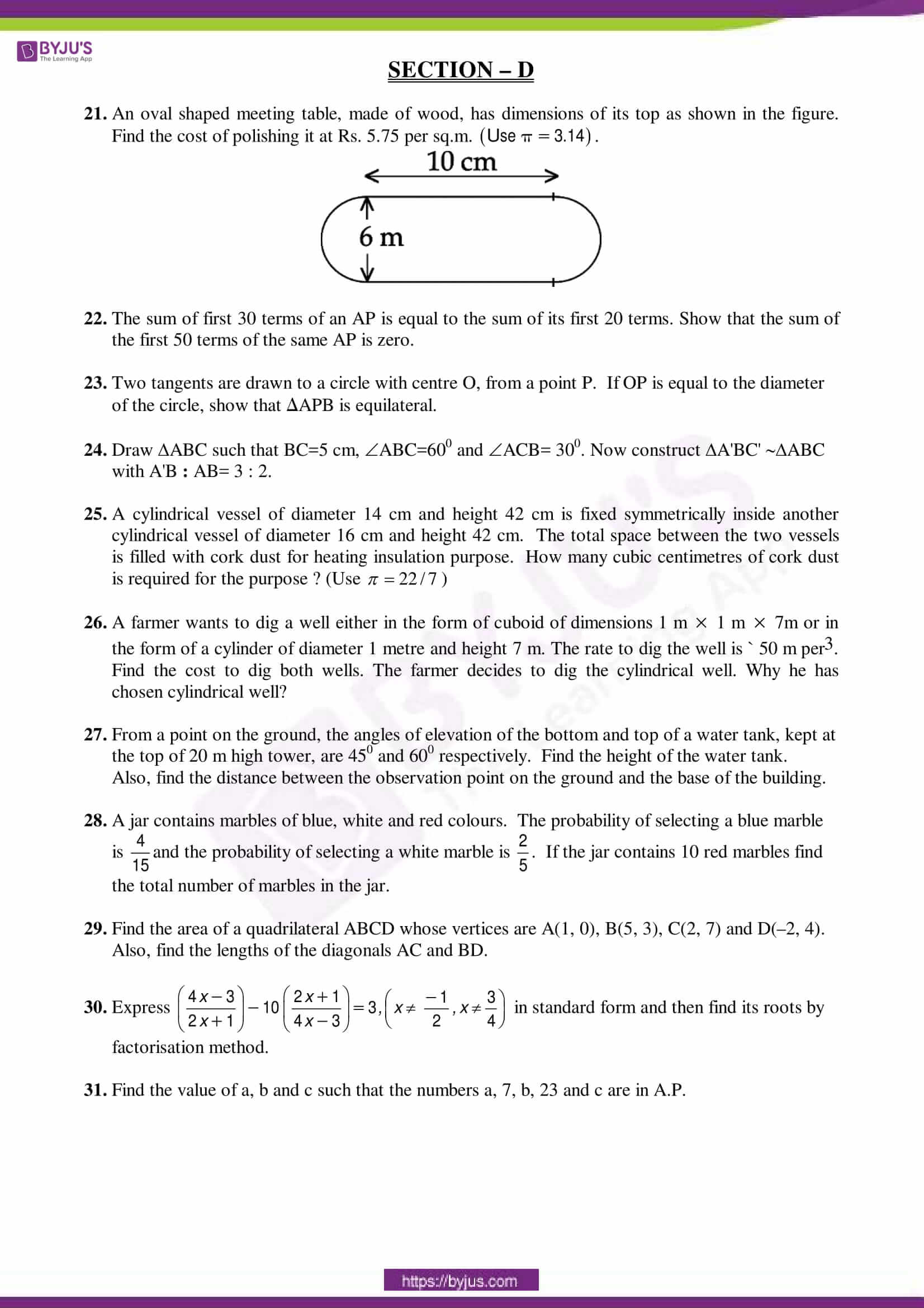# CBSE Sample Papers Class 10 SA 2 Maths Set 6

To gain command over Maths subject, it’s important that students must practice different types of Maths problems. Solving the CBSE Sample Papers Class 10 SA 2 Maths is one of the ways through which students can practice questions of varying difficulty level. So, here we are providing the CBSE Class 10 Maths Sample Paper SA 2 Set 6 in PDF format. Solving this paper will give students an idea of exam pattern and mark weightage. Also, they feel more confident while writing the exam.## Access Other Sets of CBSE Class 10 Maths SA 2 Sample Papers

Students can access the other sets of CBSE sample papers for class 10 Maths SA 2 exam from the table below.

Students can also access the latest CBSE Sample Papers for Class 10 Maths board exam at BYJU’S.

### Key Features of CBSE Sample Papers Class 10 SA 2 Maths

1. The sample papers are designed by experts.
2. These papers cover all the important topics of the syllabus.
3. Solving these sample papers will help students to understand the types of questions asked in the exam and their difficulty level.
4. Students get well versed with the exam pattern and mark distribution.
5. Practising sample papers will boost the confidence level of students.

We hope students have found this information on “CBSE Sample Papers for Class 10 SA 2 Maths Set 6” useful for their studies. To download the sample papers for all classes visit CBSE Sample Paper page. Keep learning and stay tuned for further updates on CBSE and other competitive exams. Download BYJU’S App and subscribe to YouTube channel to access interactive Maths and Science videos.

 NCERT Related Articles NCERT Solutions for Class 12 NCERT Solutions for Class 11 NCERT Solutions for Class 10 NCERT Solutions for Class 12 Maths NCERT Solutions for Class 11 Maths NCERT Solutions for Class 10 Maths NCERT Solutions for Class 12 Physics NCERT Solutions for Class 11 Physics NCERT Solutions for Class 10 Science NCERT Solutions for Class 12 Chemistry NCERT Solutions for Class 11 Chemistry NCERT Solutions for Class 6 NCERT Solutions for Class 12 Biology NCERT Solutions for Class 11 Biology NCERT Solutions for Class 6 Maths NCERT Solutions for Class 9 NCERT Solutions for Class 8 NCERT Solutions for Class 6 Science NCERT Solutions for Class 9 Maths NCERT Solutions for Class 8 Maths NCERT Solutions for Class 4 NCERT Solutions for Class 9 Science NCERT Solutions for Class 8 Science NCERT Solutions for Class 4 Maths NCERT Solutions for Class 5 NCERT Solutions for Class 7 NCERT Solutions for Class 7 Maths NCERT Solutions for Class 4 Science NCERT Solutions for Class 5 Maths NCERT Solutions for Class 7 Science NCERT Solutions NCERT Books NCERT Solutions for Class 5 Science